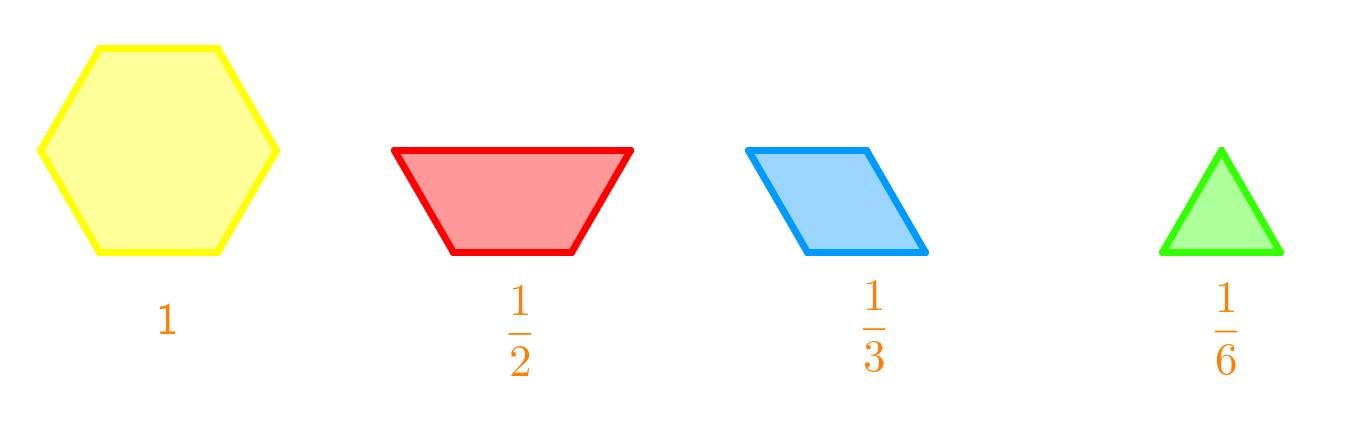# Adding and subtracting mixed numbers#### Everything You Need in One Place

Homework problems? Exam preparation? Trying to grasp a concept or just brushing up the basics? Our extensive help & practice library have got you covered.#### Learn and Practice With Ease

Our proven video lessons ease you through problems quickly, and you get tonnes of friendly practice on questions that trip students up on tests and finals.#### Instant and Unlimited Help

Our personalized learning platform enables you to instantly find the exact walkthrough to your specific type of question. Activate unlimited help now!

0/6
##### Intros
###### Lessons
1. Simplify fractions: Method A - By using greatest common factors
2. Simplify fractions: Method B - By using common factors
3. What are equivalent fractions?
5. How to subtract frations?
• subtracting with improper fractions
• subtracting with mixed numbers
6. Proper fractions VS. Improper fractions VS. Mixed fractions
0/5
##### Examples
###### Lessons
1. Subtract or add using the given diagram.1.2.1. $1\frac{3}{6}+\frac{3}{8}$
3. Maren goes to soccer practice for $2\frac{4}{5}$ hour each day. In the morning, she has $1\frac{3}{4}$ hour of practice. How many hours of practice does she have in the afternoon?
1. Hannah ran for $1\frac{1}{4}$ hour and then biked for $1\frac{2}{3}$ hour. For how long did she exercise?